# Chapter 16 Electric Energy and Capacitance Electric Potential

• Slides: 53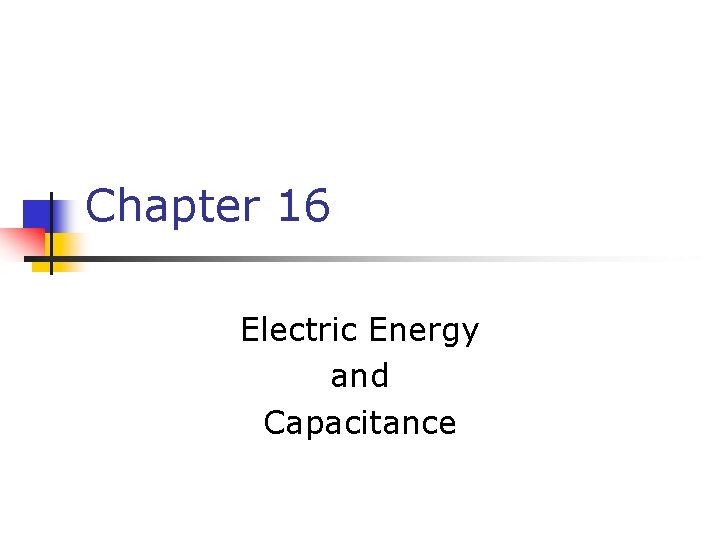Chapter 16 Electric Energy and CapacitanceElectric Potential Energy n n n The electrostatic force is a conservative force It is possible to define an electrical potential energy function with this force Work done by a conservative force is equal to the negative of the change in potential energy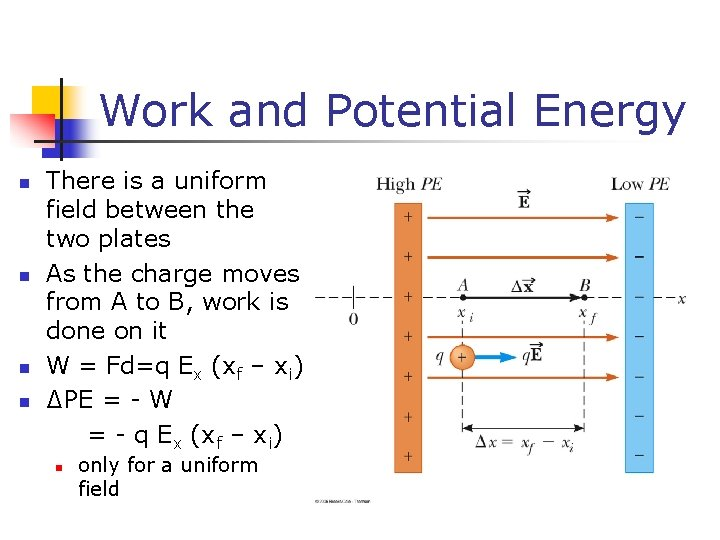Work and Potential Energy n n There is a uniform field between the two plates As the charge moves from A to B, work is done on it W = Fd=q Ex (xf – xi) ∆PE = - W = - q Ex (xf – xi) n only for a uniform field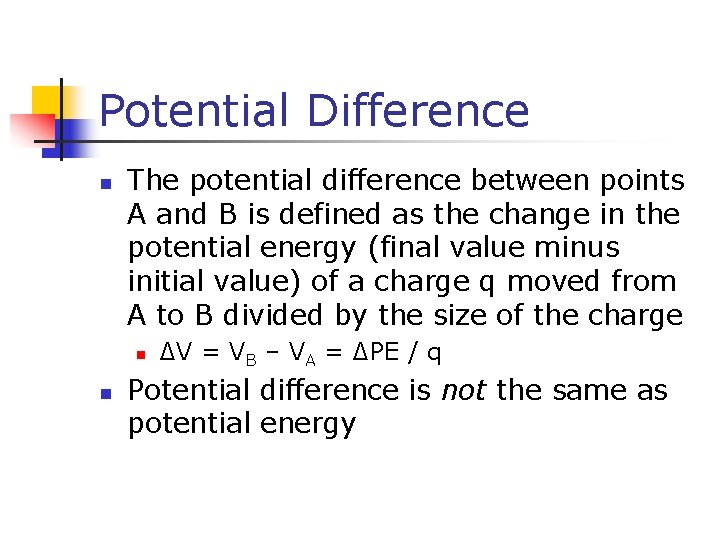Potential Difference n The potential difference between points A and B is defined as the change in the potential energy (final value minus initial value) of a charge q moved from A to B divided by the size of the charge n n ∆V = VB – VA = ∆PE / q Potential difference is not the same as potential energyPotential Difference, cont. n n n Another way to relate the energy and the potential difference: ∆PE = q ∆V Both electric potential energy and potential difference are scalar quantities Units of potential difference n n V = J/C A special case occurs when there is a uniform electric field n DV = VB – VA= -Ex Dx n Gives more information about units: N/C = V/mEnergy and Charge Movements n n A positive charge gains electrical potential energy when it is moved in a direction opposite the electric field If a charge is released in the electric field, it experiences a force and accelerates, gaining kinetic energy n n As it gains kinetic energy, it loses an equal amount of electrical potential energy A negative charge loses electrical potential energy when it moves in the direction opposite the electric field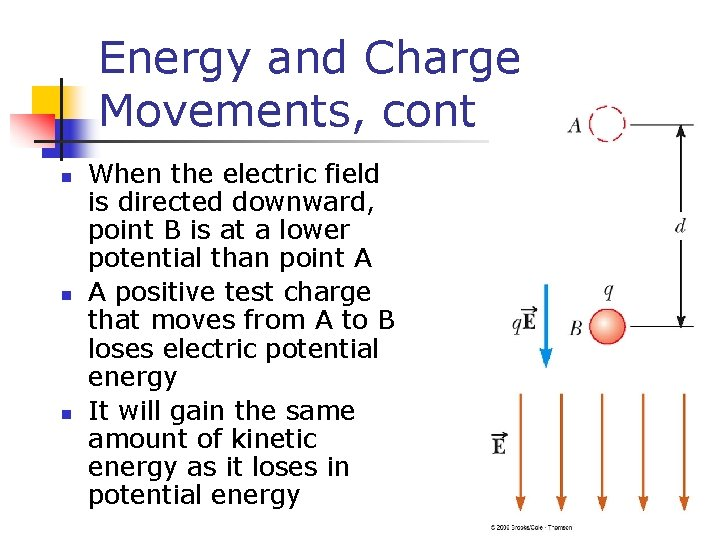Energy and Charge Movements, cont n n n When the electric field is directed downward, point B is at a lower potential than point A A positive test charge that moves from A to B loses electric potential energy It will gain the same amount of kinetic energy as it loses in potential energySummary of Positive Charge Movements and Energy n When a positive charge is placed in an electric field n n It moves in the direction of the field It moves from a point of higher potential to a point of lower potential Its electrical potential energy decreases Its kinetic energy increases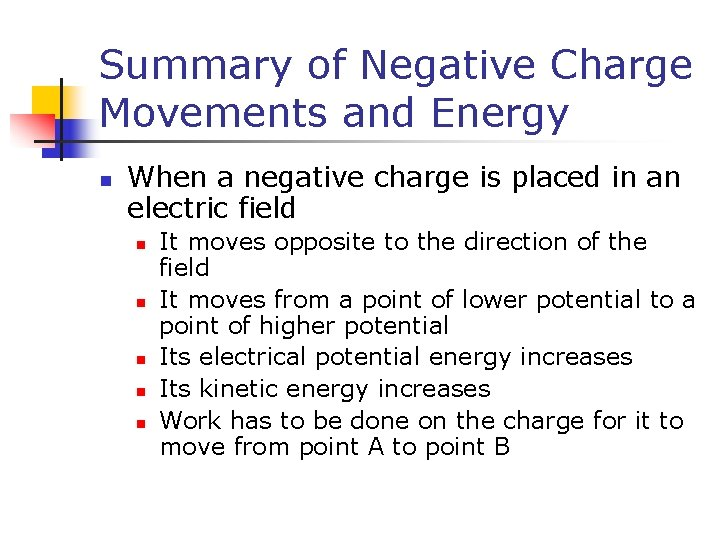Summary of Negative Charge Movements and Energy n When a negative charge is placed in an electric field n n n It moves opposite to the direction of the field It moves from a point of lower potential to a point of higher potential Its electrical potential energy increases Its kinetic energy increases Work has to be done on the charge for it to move from point A to point BElectric Potential of a Point Charge n n n The point of zero electric potential is taken to be at an infinite distance from the charge The potential created by a point charge q at any distance r from the charge is A potential exists at some point in space whether or not there is a test charge at that pointElectric Field and Electric Potential Depend on Distance n n The electric field is proportional to 1/r 2 The electric potential is proportional to 1/rElectric Potential of Multiple Point Charges n n Superposition principle applies The total electric potential at some point P due to several point charges is the algebraic sum of the electric potentials due to the individual charges n The algebraic sum is used because potentials are scalar quantities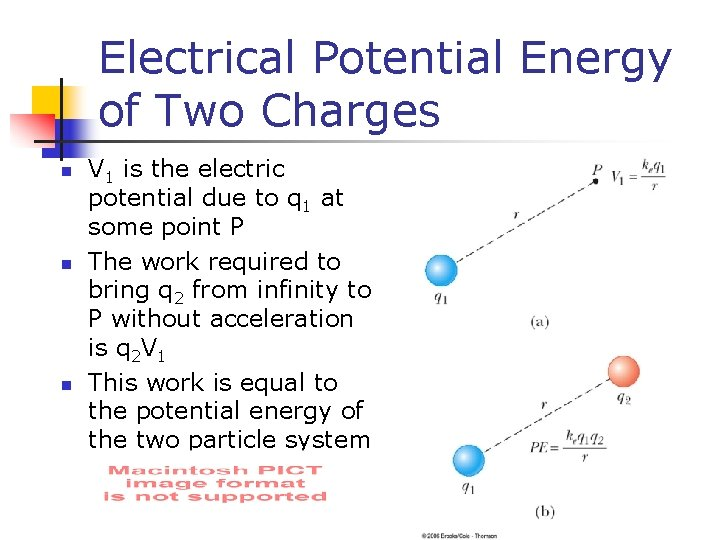Electrical Potential Energy of Two Charges n n n V 1 is the electric potential due to q 1 at some point P The work required to bring q 2 from infinity to P without acceleration is q 2 V 1 This work is equal to the potential energy of the two particle systemNotes About Electric Potential Energy of Two Charges n If the charges have the same sign, PE is positive n n n Positive work must be done to force the two charges near one another The like charges would repel If the charges have opposite signs, PE is negative n n The force would be attractive Work must be done to hold back the unlike charges from accelerating as they are brought close together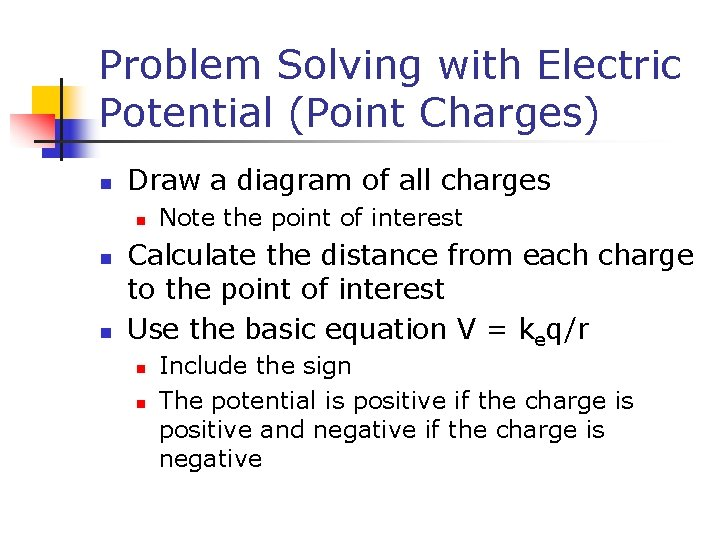Problem Solving with Electric Potential (Point Charges) n Draw a diagram of all charges n n n Note the point of interest Calculate the distance from each charge to the point of interest Use the basic equation V = keq/r n n Include the sign The potential is positive if the charge is positive and negative if the charge is negativeProblem Solving with Electric Potential, cont n Use the superposition principle when you have multiple charges n n Take the algebraic sum Remember that potential is a scalar quantity n So no components to worry about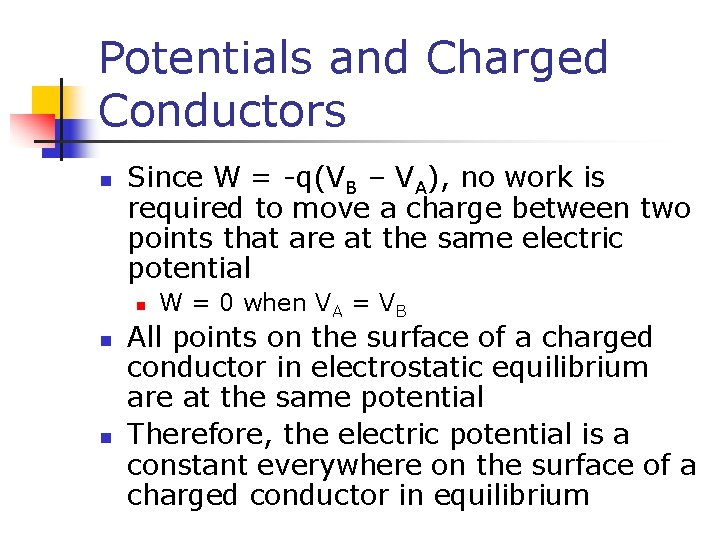Potentials and Charged Conductors n Since W = -q(VB – VA), no work is required to move a charge between two points that are at the same electric potential n n n W = 0 when VA = VB All points on the surface of a charged conductor in electrostatic equilibrium are at the same potential Therefore, the electric potential is a constant everywhere on the surface of a charged conductor in equilibrium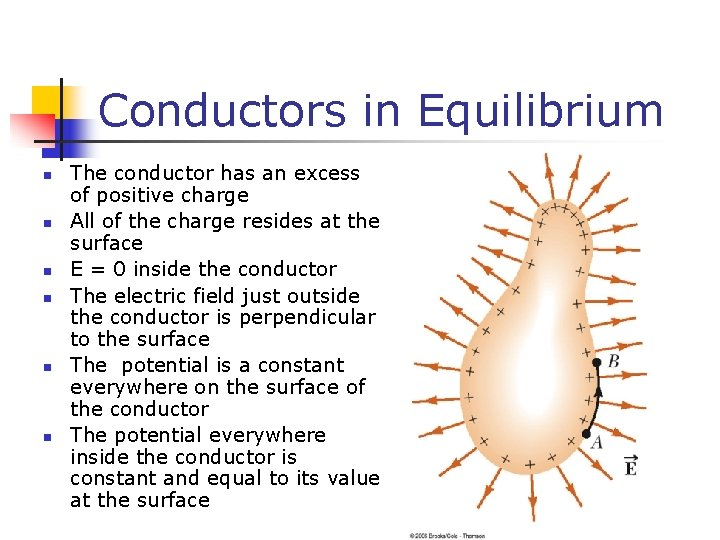Conductors in Equilibrium n n n The conductor has an excess of positive charge All of the charge resides at the surface E = 0 inside the conductor The electric field just outside the conductor is perpendicular to the surface The potential is a constant everywhere on the surface of the conductor The potential everywhere inside the conductor is constant and equal to its value at the surface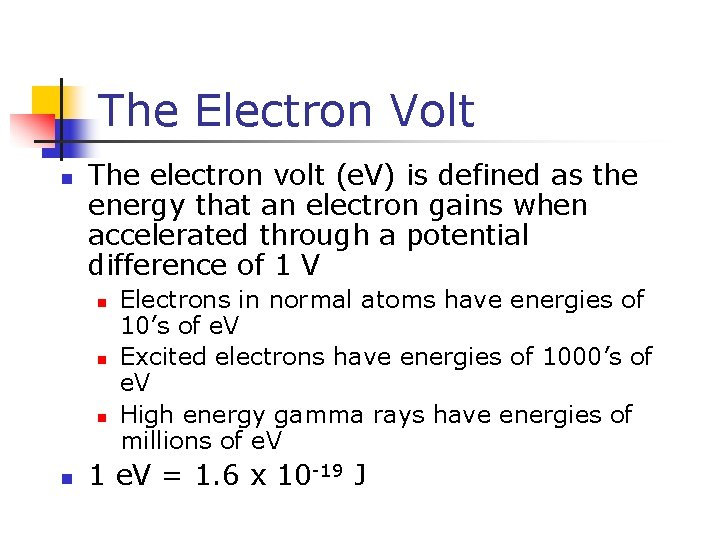The Electron Volt n The electron volt (e. V) is defined as the energy that an electron gains when accelerated through a potential difference of 1 V n n Electrons in normal atoms have energies of 10’s of e. V Excited electrons have energies of 1000’s of e. V High energy gamma rays have energies of millions of e. V 1 e. V = 1. 6 x 10 -19 J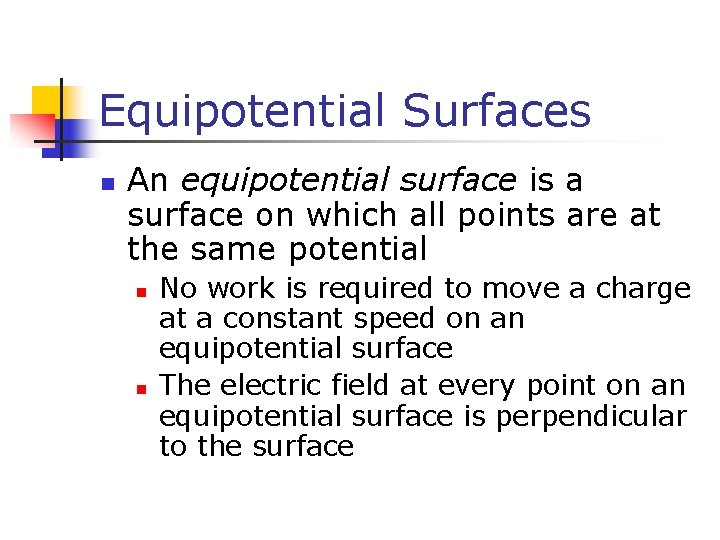Equipotential Surfaces n An equipotential surface is a surface on which all points are at the same potential n n No work is required to move a charge at a constant speed on an equipotential surface The electric field at every point on an equipotential surface is perpendicular to the surfaceEquipotentials and Electric Fields Lines – Positive Charge n n The equipotentials for a point charge are a family of spheres centered on the point charge The field lines are perpendicular to the electric potential at all points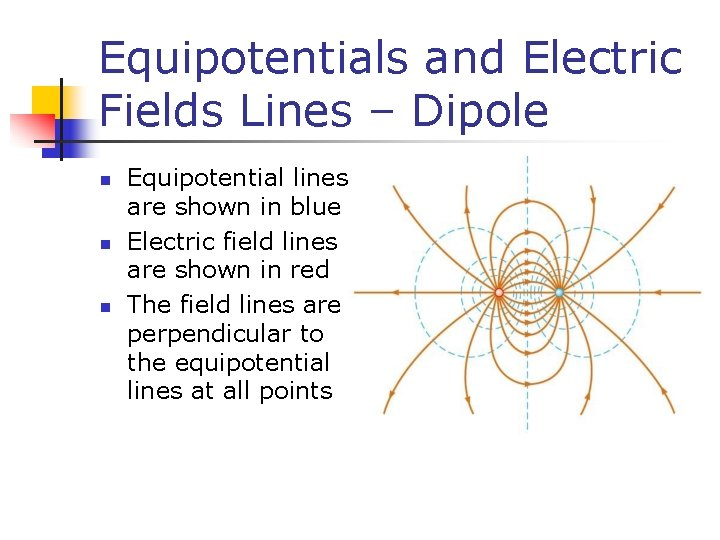Equipotentials and Electric Fields Lines – Dipole n n n Equipotential lines are shown in blue Electric field lines are shown in red The field lines are perpendicular to the equipotential lines at all points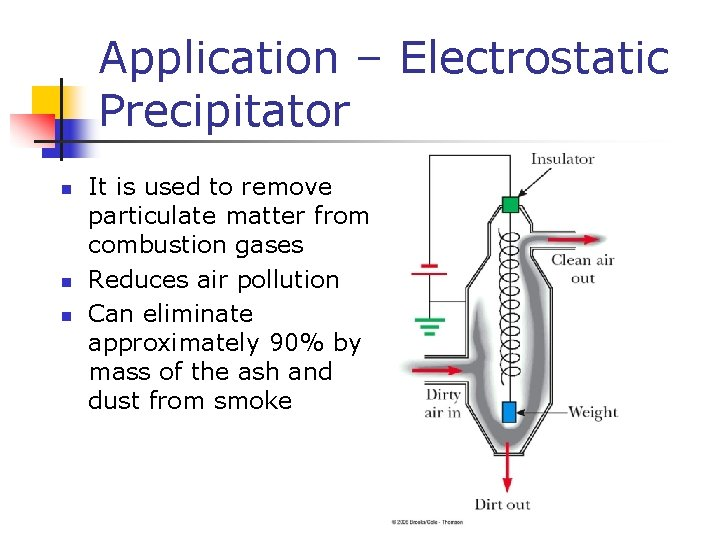Application – Electrostatic Precipitator n n n It is used to remove particulate matter from combustion gases Reduces air pollution Can eliminate approximately 90% by mass of the ash and dust from smokeApplication – Electrostatic Air Cleaner n n Used in homes to relieve the discomfort of allergy sufferers It uses many of the same principles as the electrostatic precipitator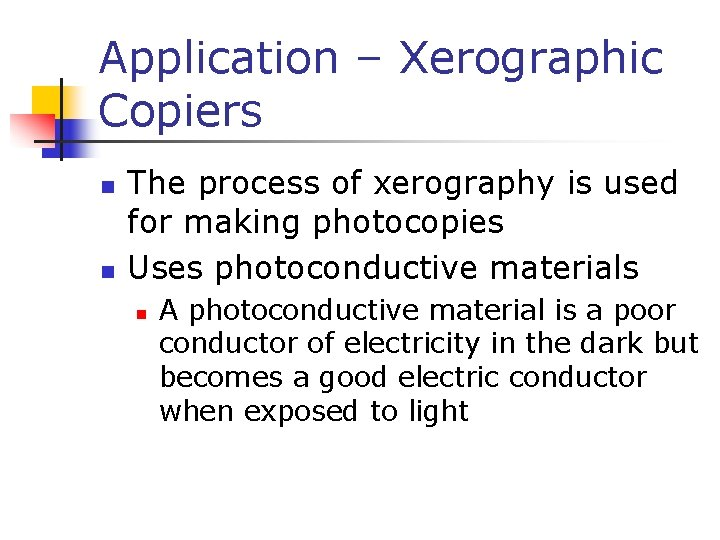Application – Xerographic Copiers n n The process of xerography is used for making photocopies Uses photoconductive materials n A photoconductive material is a poor conductor of electricity in the dark but becomes a good electric conductor when exposed to lightThe Xerographic ProcessApplication – Laser Printer n The steps for producing a document on a laser printer is similar to the steps in the xerographic process n n Steps a, c, and d are the same The major difference is the way the image forms on the selenium-coated drum n n n A rotating mirror inside the printer causes the beam of the laser to sweep across the seleniumcoated drum The electrical signals form the desired letter in positive charges on the selenium-coated drum Toner is applied and the process continues as in the xerographic processCapacitance n n A capacitor is a device used in a variety of electric circuits The capacitance, C, of a capacitor is defined as the ratio of the magnitude of the charge on either conductor (plate) to the magnitude of the potential difference between the conductors (plates)Capacitance, cont n n Units: Farad (F) n n 1 F=1 C/V A Farad is very large n Often will see µF or p. F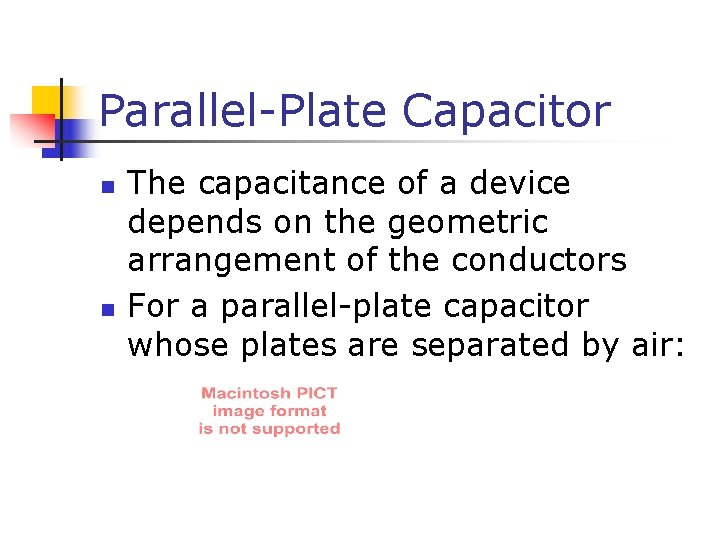Parallel-Plate Capacitor n n The capacitance of a device depends on the geometric arrangement of the conductors For a parallel-plate capacitor whose plates are separated by air:Parallel-Plate Capacitor, Example n n n The capacitor consists of two parallel plates Each have area A They are separated by a distance d The plates carry equal and opposite charges When connected to the battery, charge is pulled off one plate and transferred to the other plate The transfer stops when DVcap = DVbatteryElectric Field in a Parallel. Plate Capacitor n The electric field between the plates is uniform n n n Near the center Nonuniform near the edges The field may be taken as constant throughout the region between the platesApplications of Capacitors – Camera Flash n The flash attachment on a camera uses a capacitor n n n A battery is used to charge the capacitor The energy stored in the capacitor is released when the button is pushed to take a picture The charge is delivered very quickly, illuminating the subject when more light is needed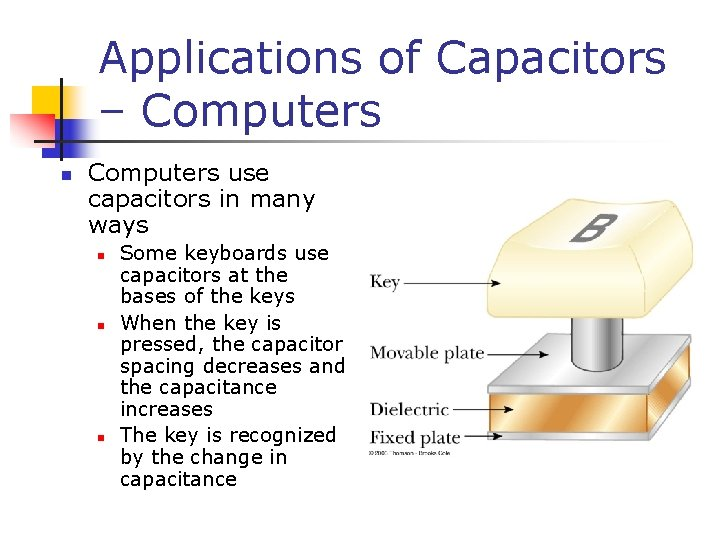Applications of Capacitors – Computers n Computers use capacitors in many ways n n n Some keyboards use capacitors at the bases of the keys When the key is pressed, the capacitor spacing decreases and the capacitance increases The key is recognized by the change in capacitance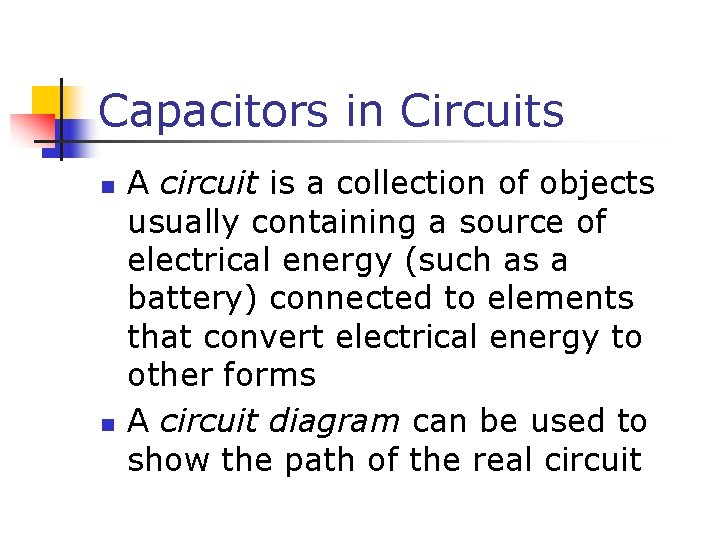Capacitors in Circuits n n A circuit is a collection of objects usually containing a source of electrical energy (such as a battery) connected to elements that convert electrical energy to other forms A circuit diagram can be used to show the path of the real circuit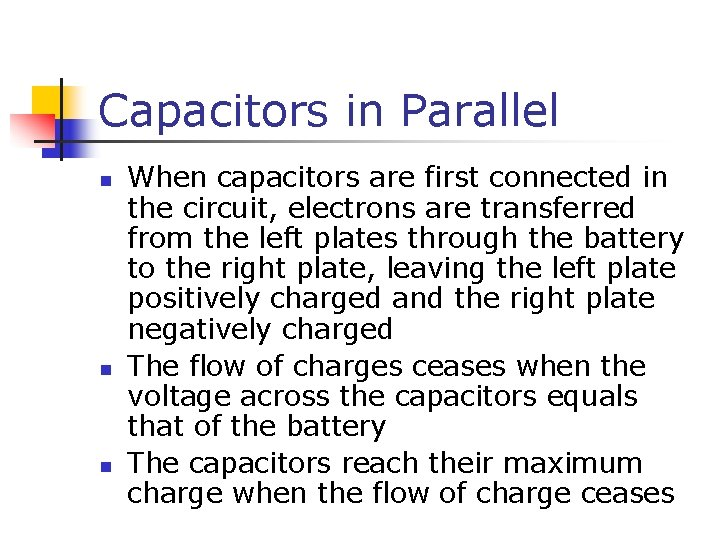Capacitors in Parallel n n n When capacitors are first connected in the circuit, electrons are transferred from the left plates through the battery to the right plate, leaving the left plate positively charged and the right plate negatively charged The flow of charges ceases when the voltage across the capacitors equals that of the battery The capacitors reach their maximum charge when the flow of charge ceases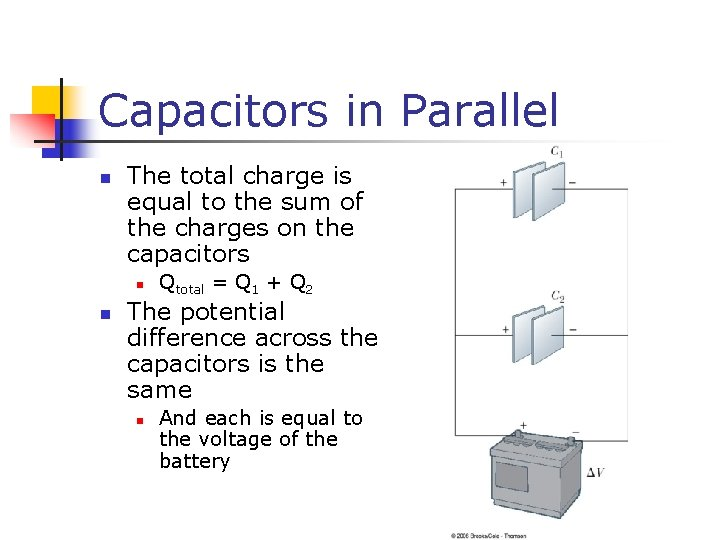Capacitors in Parallel n The total charge is equal to the sum of the charges on the capacitors n n Qtotal = Q 1 + Q 2 The potential difference across the capacitors is the same n And each is equal to the voltage of the batteryMore About Capacitors in Parallel n The capacitors can be replaced with one capacitor with a capacitance of Ceq n The equivalent capacitor must have exactly the same external effect on the circuit as the original capacitorsCapacitors in Parallel, final n n Ceq = C 1 + C 2 + … The equivalent capacitance of a parallel combination of capacitors is greater than any of the individual capacitorsCapacitors in Series n n n When a battery is connected to the circuit, electrons are transferred from the left plate of C 1 to the right plate of C 2 through the battery As this negative charge accumulates on the right plate of C 2, an equivalent amount of negative charge is removed from the left plate of C 2, leaving it with an excess positive charge All of the right plates gain charges of –Q and all the left plates have charges of +QMore About Capacitors in Series n n An equivalent capacitor can be found that performs the same function as the series combination The potential differences add up to the battery voltageCapacitors in Series, cont n n The equivalent capacitance of a series combination is always less than any individual capacitor in the combination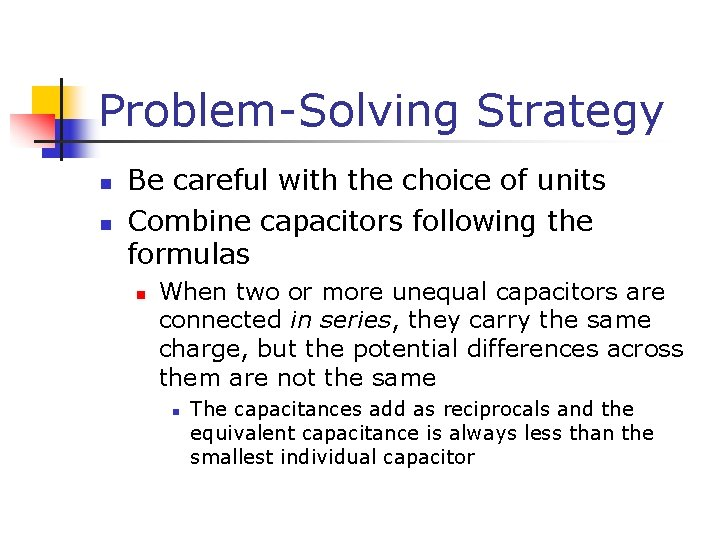Problem-Solving Strategy n n Be careful with the choice of units Combine capacitors following the formulas n When two or more unequal capacitors are connected in series, they carry the same charge, but the potential differences across them are not the same n The capacitances add as reciprocals and the equivalent capacitance is always less than the smallest individual capacitor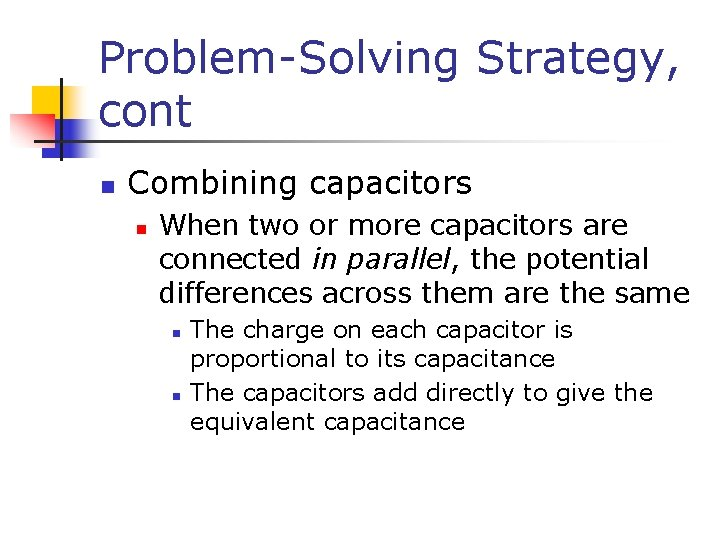Problem-Solving Strategy, cont n Combining capacitors n When two or more capacitors are connected in parallel, the potential differences across them are the same n n The charge on each capacitor is proportional to its capacitance The capacitors add directly to give the equivalent capacitance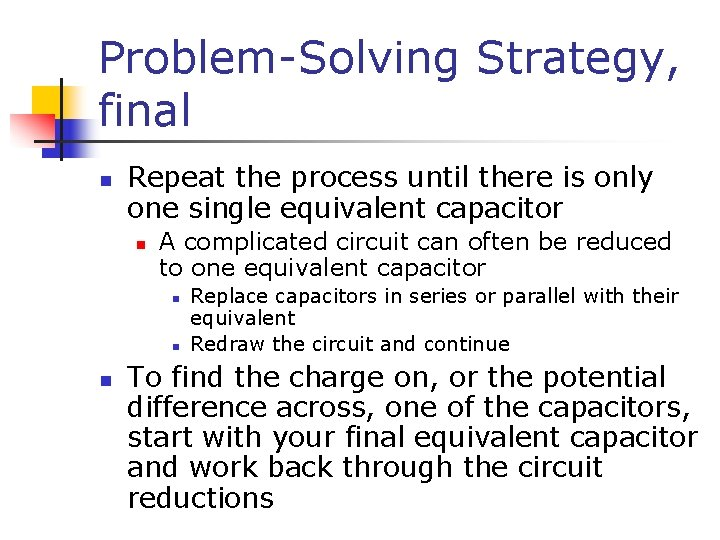Problem-Solving Strategy, final n Repeat the process until there is only one single equivalent capacitor n A complicated circuit can often be reduced to one equivalent capacitor n n n Replace capacitors in series or parallel with their equivalent Redraw the circuit and continue To find the charge on, or the potential difference across, one of the capacitors, start with your final equivalent capacitor and work back through the circuit reductionsProblem-Solving Strategy, Equation Summary n Use the following equations when working through the circuit diagrams: n n n Capacitance equation: C = Q / DV Capacitors in parallel: Ceq = C 1 + C 2 + … Capacitors in parallel all have the same voltage differences as does the equivalent capacitance Capacitors in series: 1/Ceq = 1/C 1 + 1/C 2 + … Capacitors in series all have the same charge, Q, as does their equivalent capacitance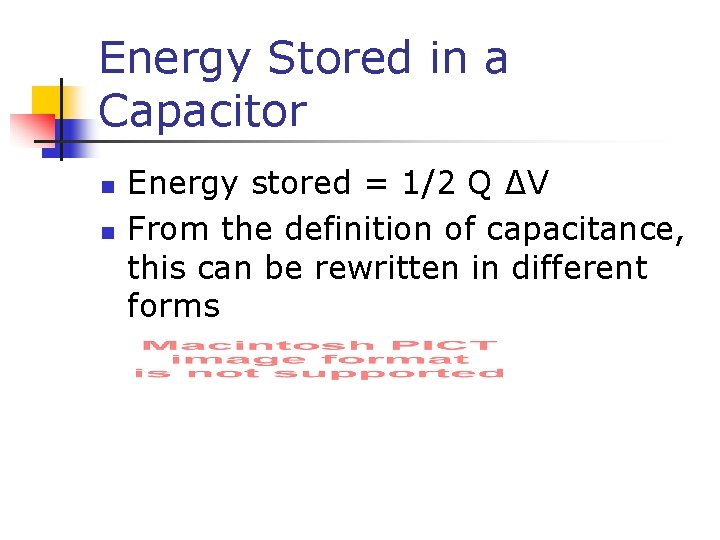Energy Stored in a Capacitor n n Energy stored = 1/2 Q ∆V From the definition of capacitance, this can be rewritten in different formsApplications n Defibrillators n n n When fibrillation occurs, the heart produces a rapid, irregular pattern of beats A fast discharge of electrical energy through the heart can return the organ to its normal beat pattern In general, capacitors act as energy reservoirs that can slowly charged and then discharged quickly to provide large amounts of energy in a short pulseCapacitors with Dielectrics n A dielectric is an insulating material that, when placed between the plates of a capacitor, increases the capacitance n n Dielectrics include rubber, plastic, or waxed paper C = KCo = K o(A/d) n The capacitance is multiplied by the factor K when the dielectric completely fills the region between the platesCapacitors with DielectricsDielectric Strength n n For any given plate separation, there is a maximum electric field that can be produced in the dielectric before it breaks down and begins to conduct This maximum electric field is called the dielectric strengthAn Atomic Description of Dielectrics n n Polarization occurs when there is a separation between the “centers of gravity” of its negative charge and its positive charge In a capacitor, the dielectric becomes polarized because it is in an electric field that exists between the plates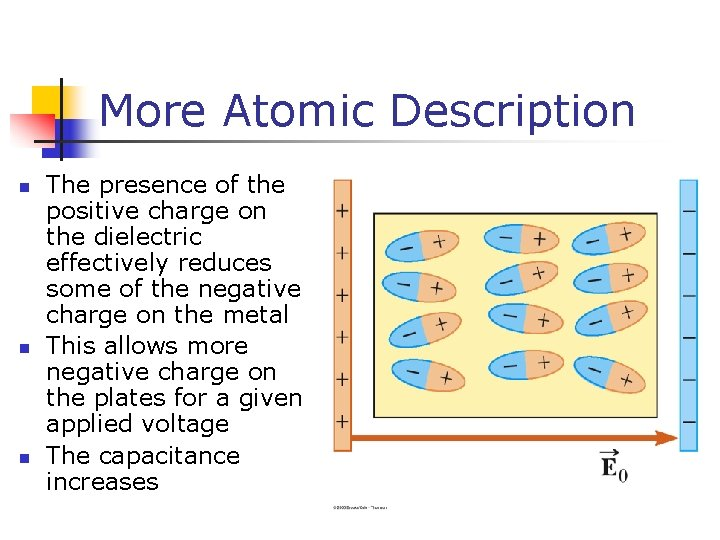More Atomic Description n The presence of the positive charge on the dielectric effectively reduces some of the negative charge on the metal This allows more negative charge on the plates for a given applied voltage The capacitance increases